# Logical Reasoning for MBA exam - Set 2

1)   In the question below is given a statement followed by two courses of action numbered I and II. You have to assume everything in the statement to be true and based on the information given in the statement, decide which of the suggested courses of action logically follow(s) for pursuing.

(A) If only I follows
(B) If only II follows
(C) If either I or II follows
(D) If neither I nor II follow
(E) If both I and II follow.

Statement - Many students have gone on a mass leave in protest against the institute’s new policies.

Courses of Action - I. The Institute should immediately withdraw the new policies.

II. All these students should be suspended.

a. Only I follows
b. Only II follows
c. Either I or II follows
d. Neither I nor II follow
e. Both I and II follow
 Answer  Explanation ANSWER: Neither I nor II follow Explanation: Here, drastic measures such as getting rid of the policies or suspending all students, will not help. Thus, neither I nor II follows.

2)   At what time between 4 and 4.59 will the hands of a watch point in opposite directions?

a. 45 min. past 4
b. 40 min. past 4
c. 50 (4/11) min. past 4
d. 54 (6/11) past 4
 Answer  Explanation ANSWER: 54 (6/11) past 4 Explanation: At 4 o'clock, the hands of the watch are 20 min. spaces apart.To be in opposite directions, they must be 30 min. spaces apart.Minute hand must gain 50 min. spaces.55 min. spaces are gained in 60 min.50 min. spaces are gained in 60/55 x 50 = 54 (6/11) minRequired time = 54 6/11 min past 4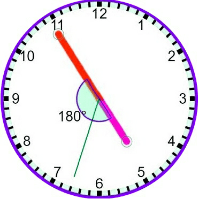3)   At what time between 7 and 8 will the hands of a clock be in the same straight line but, not together?

a. 5 (5/11) min past 7
b. 5 (4/11) min past 7
c. 5 (3/11) min past 7
d. 5 (2/11) min past 7
 Answer  Explanation ANSWER: 5 (5/11) min past 7 Explanation: When the hands of the clock are in the same straight line but not together, they are 30 minute spaces apart.At 7 o'clock, they are 25 min. spaces apart.Minute hand must gain only 5 min. space.55 min. spaces are gained in 60 min.5 min. spaces would be gained in (60/55) x 5 = 5 (5/11) minRequired time = 5 (5/11) min past 7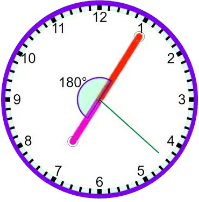4)   The question given below has a set of three or four statements. Each set of statements is further divided into three segments. Choose the alternative where the third segment in the statement can be logically deduced using both the preceding two, but not just from one of them.

Statement - I. All bats are lions.
II. No cow is lion.
III. Some camels are cows.

Conclusion - I. Some lions are camels.
II. No camel is bat.
III. Some bats are cows.

a. Only I follows
b. Only II follows
c. Only III follows
d. Only I and II follows
e. None of these
 Answer  Explanation ANSWER: None of these Explanation: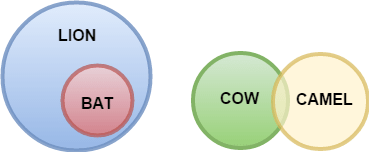5)   The question given below has a set of three or four statements. Each set of statements is further divided into three segments. Choose the alternative where the third segment in the statement can be logically deduced using both the preceding two, but not just from one of them.

Statement - I. Some pens are books.
II. All temples are books.
III. Some libraries are temples.

Conclusion - I. Some libraries are pens.
II. Some pens are temples.
III. Some libraries are books.

a. Only I follows
b. Only II follows
c. None follows
d. Only I and II follows
e. Both II and III follow
 Answer  Explanation ANSWER: None follows Explanation: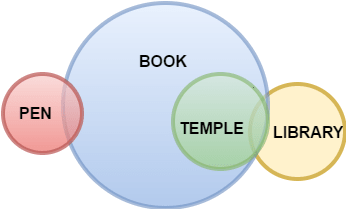6)   The question given below has a set of three or four statements. Each set of statements is further divided into three segments. Choose the alternative where the third segment in the statement can be logically deduced using both the preceding two, but not just from one of them.

Statement - I. All flowers are toys.
II. Some toys are idiots.
III. Some angels are idiots.

Conclusion - I. Some angels are toys.
II. Some idiots are flowers.
III. Some flowers are angels.

a. Only I follows
b. Only II follows
c. Only III follows
d. Only I and II follows
e. None follows
 Answer  Explanation ANSWER: None follows Explanation: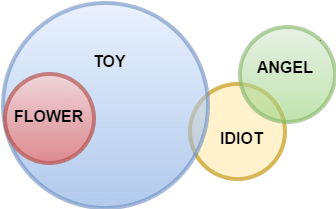7)   The question given below has a set of three or four statements. Each set of statements is further divided into three segments. Choose the alternative where the third segment in the statement can be logically deduced using both the preceding two, but not just from one of them.

Statement - I. All fruits are lions
II. All lions are foxes
III. Some foxes are beggars

Conclusion - I. All fruits are foxes.
II. Some fruits are beggars.

a. Only I follows
b. Only II follows
c. Only III follows
d. Only I and II follows
e. Both II and III follow
 Answer  Explanation ANSWER: Only I follows Explanation: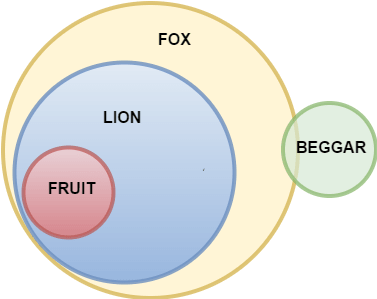8)   The question given below has a set of three or four statements. Each set of statements is further divided into three segments. Choose the alternative where the third segment in the statement can be logically deduced using both the preceding two, but not just from one of them.

Statement- I. No key is door.
II. All doors are pens.
III. Some pens are houses.

Conclusion - I. No key is house.
II. Some pens are doors.

a. Only I follows
b. Only II follows
c. Only III follows
d. Only I and II follows
e. Both II and III follow
 Answer  Explanation ANSWER: Only II follows Explanation: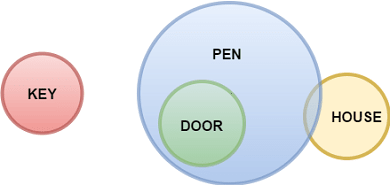9)   The following question consists of some Problem Figures followed by other figures marked 1, 2, 3, 4 called the Answer Figures.

Find out the correct answer figure that should come next in the sequence of problem figures.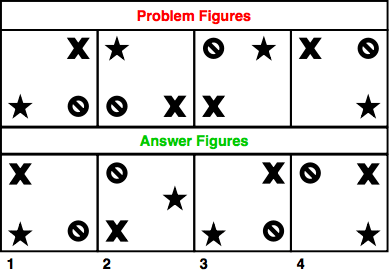a. 1
b. 2
c. 3
d. 4
 Answer  Explanation ANSWER: 3 Explanation: The elements are moved forward in a clock-wise direction by single step each time. Thus, the answer figure should be figure 3.

10)   The following question consists of some Problem Figures followed by other figures marked 1, 2, 3, 4 called the Answer Figures.

Find out the correct answer figure that should come next in the sequence of problem figures.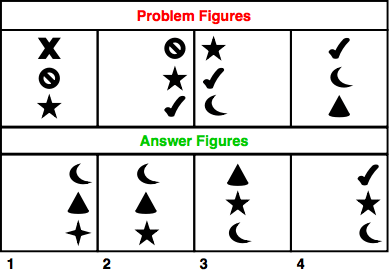a. 1
b. 2
c. 3
d. 4
 Answer  Explanation ANSWER: 1 Explanation: 2nd element becomes the top element in the next figure. Third element becomes second element. The previous element is deleted and replaced with another.The complete column of figures moves one-third step forward each time. Thus, the answer figure should be figure 1.

11)   The following question consists of some Problem Figures followed by other figures marked 1, 2, 3, 4 called the Answer Figures.

Find out the correct answer figure that should come next in the sequence of problem figures.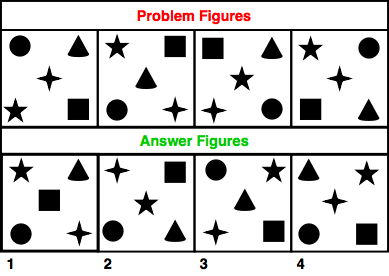a. 1
b. 2
c. 3
d. 4
 Answer  Explanation ANSWER: 3 Explanation: The circle and square move in anti-clockwise direction in a set order.The elements in the centre follow a set order as - 4 - pointed star, triangle, 5-pointed star, 4-pointed star and so on.Thus, the answer figure should be figure 3.

12)   The following question consists of some Problem Figures followed by other figures marked 1, 2, 3, 4 called the Answer Figures.

Find out the correct answer figure that should come next in the sequence of problem figures.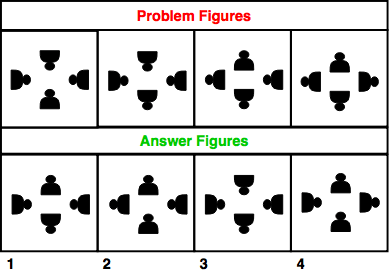a. 1
b. 2
c. 3
d. 4
 Answer  Explanation ANSWER: 4 Explanation: The element at the bottom is rotated through 180 degrees.Then, two elements in the clockwise direction are rotated through 180 degrees.In the third step, the element to the right is rotated through 180 degrees.Thus, the next should be rotation of next two elements in the clockwise direction should be rotated by 180 degrees.The answer figure should be figure 4.

13)   The following question consists of some Problem Figures followed by other figures marked 1, 2, 3, 4 called the Answer Figures.

Find out the correct answer figure that should come next in the sequence of problem figures.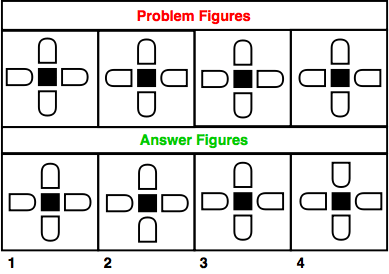a. 1
b. 2
c. 3
d. 4
 Answer  Explanation ANSWER: 1 Explanation: The odd numbered figures are identical and the even numbered figures are identical. Thus, in the same order, the answer figure should be figure 1.

14)   The following question consists of some Problem Figures followed by other figures marked 1, 2, 3, 4 called the Answer Figures.

Find out the correct answer figure that should come next in the sequence of problem figures.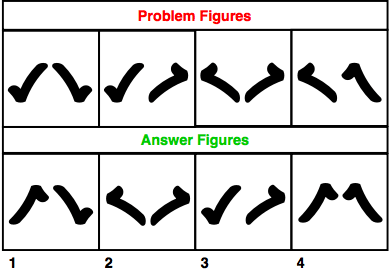a. 1
b. 2
c. 3
d. 4
 Answer  Explanation ANSWER: 4 Explanation: The elements are mirror-images in the first step. In the next step, element on the right is rotated and in the next step, mirror-image is obtained. Similarly, in the next step, element on the right is rotated and the next figure should be the mirror-image. Thus, the answer figure should be figure 4.

15)   The following question consists of some Problem Figures followed by other figures marked 1, 2, 3, 4 called the Answer Figures.

Find out the correct answer figure that should come next in the sequence of problem figures.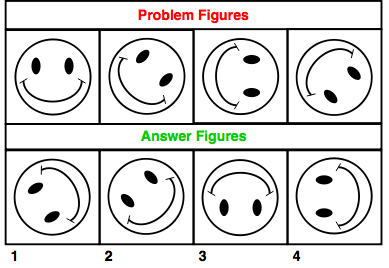a. 1
b. 2
c. 3
d. 4
 Answer  Explanation ANSWER: 3 Explanation: The figure is rotated through 45 degrees in each step, i.e. 0, 45, 90, 135, 180 and so on.Thus, the answer figure should be figure 3.